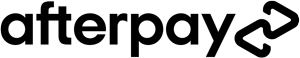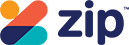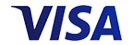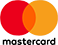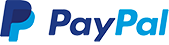Grid List
WAS \$9.95 NOW \$4.95
Increase value Decrease value
WAS \$9.95 NOW \$4.95
Increase value Decrease value
WAS \$9.95 NOW \$4.95
Increase value Decrease value
WAS \$9.95 NOW \$4.95
Increase value Decrease value
WAS \$9.95 NOW \$4.95
Increase value Decrease value
WAS \$9.95 NOW \$4.95
Increase value Decrease value
WAS \$9.95 NOW \$4.95
Increase value Decrease value
WAS \$9.95 NOW \$4.95
Increase value Decrease value
WAS \$9.95 NOW \$4.95
Increase value Decrease value
WAS \$9.95 NOW \$4.95
Increase value Decrease value
WAS \$9.95 NOW \$4.95
Increase value Decrease value
WAS \$9.95 NOW \$4.95
Increase value Decrease value